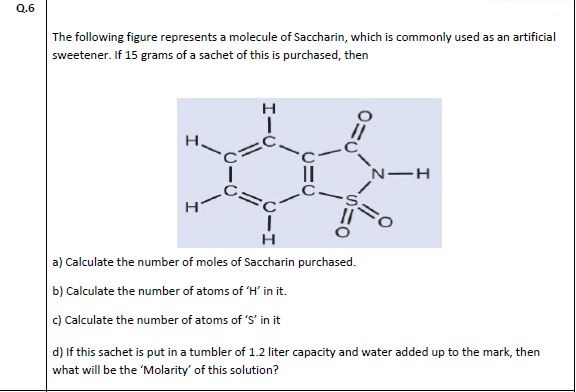# The following figure represents a molecule of Saccharin, which is commonly used as an artificia sweetener. If 15 grams of a sachet of this is purchased, then н II н a) Calculate the number of moles of Saccharin purchased. b) Calculate the number of atoms of 'H' in it. c) Calculate the number of atoms of 'S' in it d) If this sachet is put in a tumbler of 1.2 liter capacity and water added up to the mark, then what will be the 'Molarity' of this solution?

Questionhelp_outlineImage TranscriptioncloseThe following figure represents a molecule of Saccharin, which is commonly used as an artificia sweetener. If 15 grams of a sachet of this is purchased, then н II н a) Calculate the number of moles of Saccharin purchased. b) Calculate the number of atoms of 'H' in it. c) Calculate the number of atoms of 'S' in it d) If this sachet is put in a tumbler of 1.2 liter capacity and water added up to the mark, then what will be the 'Molarity' of this solution? fullscreen

1 Rating

### Want to see this answer and more?

Experts are waiting 24/7 to provide step-by-step solutions in as fast as 30 minutes!*

*Response times may vary by subject and question complexity. Median response time is 34 minutes for paid subscribers and may be longer for promotional offers.
Tagged in
Science
Chemistry

### Solutions